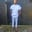Related Tags

clojure
community creator

# The neg method in ClojureChinweuba Elijah Azubuike

### The neg method

The neg method is a number test method used to test if a number is less than zero. It only accepts one numeric parameter. The neg method returns true for inputs less than zero and false for inputs greater than or equal to zero. The code line below shows the syntax for the neg method.

(neg? number)
Here is how we can use the neg method.

The neg function is particularly useful when the user wishes to confirm if their calculations did not yield a negative value. It can promptly confirm if a number variable stores a value that is less than zero.

### Code example

(ns clojure.examples.hello
(:gen-class))

(defn negg []
(def x (neg? 0))
(println x)

(def x (neg? -1))
(println x)

(def x (neg? 9))
(println x))
(negg)
Hit run to see results from the neg method. Try changing the value of the numbers being passed to the neg function and observe the changes in output.

### Code explanation

• Lines 4–12: We define a function negg.
• Line 5: We pass 0 to the neg method.
• Line 6: We print the result calculated in line 5.

Notice that neg returns false for the input 0 since 0 is non-negative.

• Line 8: We pass -1 to the neg method.
• Line 9: We print the result calculated in line 8. The result we get by passing -1 to the neg method is true.
• Line 11: We pass 9 to the neg method.
• Line 12: We print the result calculated in line 12. The result we get by passing 9 to neg is false.
• Line 13: We call the function negg defined in lines 4–12.

RELATED TAGS

clojure
community creator

CONTRIBUTORChinweuba Elijah Azubuike
RELATED COURSES

View all Courses

Keep Exploring

Learn in-demand tech skills in half the time